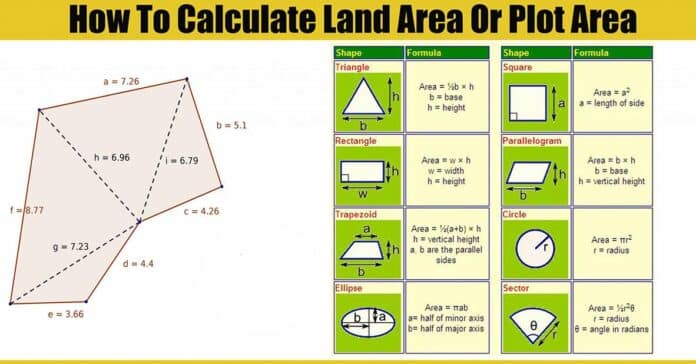# How to Calculate Land Area or Plot Area | Regular & Irregular | Irregular Land Area Calculator | Land Area Calculation Formula

In this Article today we will talk about the How to Calculate Land Area | How to Calculate Plot Area | Irregular Land Area Calculator | Land Area Calculation Formula | Land Measurement Formula | How To Measure Irregular Land Area

## How to Calculate Land Area or Plot Area:

In this post, we are going to see how to measure a piece of land at the site using the geometric method. Land Area Calculation Formula

As you know land measurement is the basic and first thing when comes to any construction. Making minor mistake will lead to heavy loss of our budget. At big sites, there may be someone who will take this hard part.

As a civil engineer, we have the responsibility to understand those basics too. Let start with the method of calculation. The Actual site may be in any shape so according to the need, we will use any of the below methods. Land Area Calculation Formula

## Method of Calculating Land Area:

• Geometric Method
• Graph Paper Method
• Plain Table Survey Method
• Theodolite Survey Method
• Using GPS Methods
• Aerial Photographs

FYI, we are not going to discuss these methods. For the sake of simplicity, we are going to discuss only geometric method now. Irregular Land Area Calculator

## Geometric Method:

Assuming that you are going to calculate the land area for a small site.

This is the basic method we used at the site. Just understand the shape of the area and start to measure and record as we do for any measurements like length, breadth etc.

We have already published Basic Area Formulas Post for your reference. So using the right shaped formula we can calculate the area of the site quickly.

## 1) Triangular Plots or Land:

These types of land are rarely found, but it does not mean that you will not have to face this problem. you can probably get these types of land at the corner of road edge or turning point. so let us solve shortly these types of problem.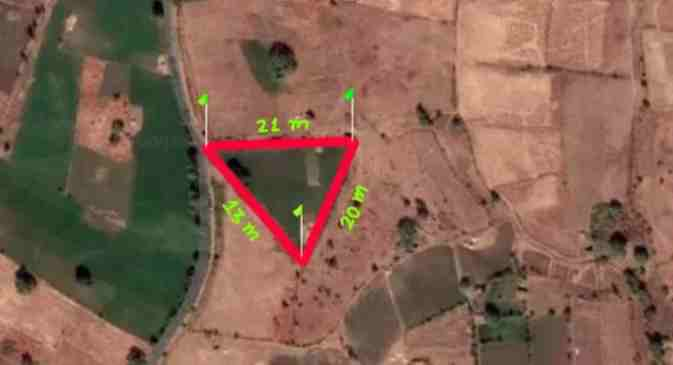### Q) Calculate the area of given triangular land having side 21 m, 13 m, and 20 m respectively.

Solution: Irregular Land Area Calculator

Let’s given sides of Triangle be,

AB = a = 20m

BC = b = 21m

CA = c = 13m

We have, Formula

As we know that, Area of a Scalene Triangle in terms of three sides,

Area of a Triangle = Area = A = √s(s-a)(s-b)(s-c)

where, s = semi-perimeter , a = length of 1st side, b = Length of 2nd side, c = Length of 3rd side

so at first calculate semi-perimeter s = (a+b+c)/2 = (20+21+13)/2 = 27m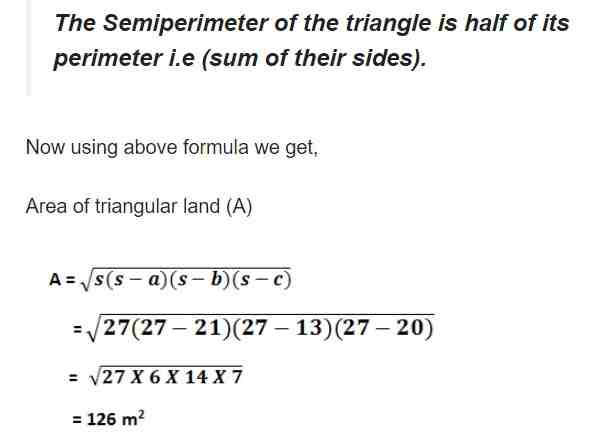Hence, in this way we can calculate area of land which are in triangular shape. now let us calculate area of rectangular plots of land. Land Measurement Formula

## 2) Rectangular Plots or Land:

These types of land are often found in all areas. The sides of lar d whose one side is equal to their opposite side and another side is equal to another side are known as rectangular land. Land Measurement Formula

It’s all sides are perpendicular to each other 1.e 90 degrees with each other. Let’s understand with the help of numerical and figure given below.

### Q) Calculate the area of rectangular land having dimensions given in the figure below: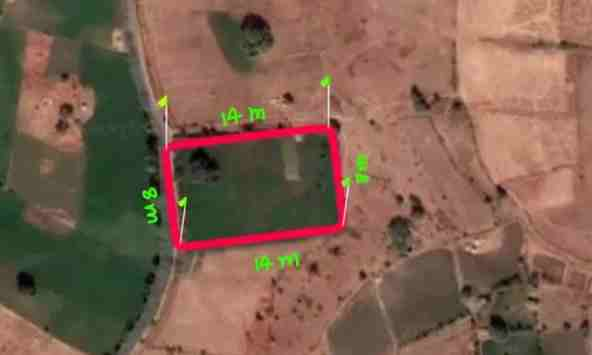Solution, Land Measurement Formula

Here in the figure two sides are 14 meter and two side are 8 meter.

so, let Length (L)= 14 meter and Breadth (B) = 8 meter

We have, Formula

Area of a Rectangular Land = A = L x B

Where, L = Length of side, B = Breadth of Side

So, Using above formula we get,

Area = L XB= (14 X 8) = 112 m2

Hence, in this way we can calculate area of land which are in rectangular shape. now let us calculate area of square plots or land. Land Measurement Formula

## 3) Square Plots or Land:

These types of land are also common in all areas. you w get the shape of and mostly and nearly in rectangular or in square shape. The sides of land whose all sides are equal to each other are known as square land. How To Measure Irregular Land Area

It’s all sides are perpendicular to each other i.e 90 degrees with each other. Let’s understand with the help of numerical and figure given below.

### a) Calculate the area of square land having dimensions given in the figure below:

Here in the figure have all sides equal and 8meter.

So, let Length (L) = 8 meter

We have, Formula

Area = L2

Where L = Length of side

Using above formula,

Area of Land (A) = (8 X 8) m2= 64 m2

Hence, in this way, we can calculate the area of land which is in square in shape. now let us calculate the area of trapezium plots or land. How To Measure Irregular Land Area

## 4) Trapezium land:

This type of land may be regular or may be irregular. I am describing here all types of land with examples. let us discuss all land with the help of figure and formula to calculate it.

### A) Land having two side parallel to each other:

These types are land is found at the side of the road edge and maybe made artificially for a different purpose. In this type of land two s des are parallel to each other but the other two sides are not. Let us understand with an example.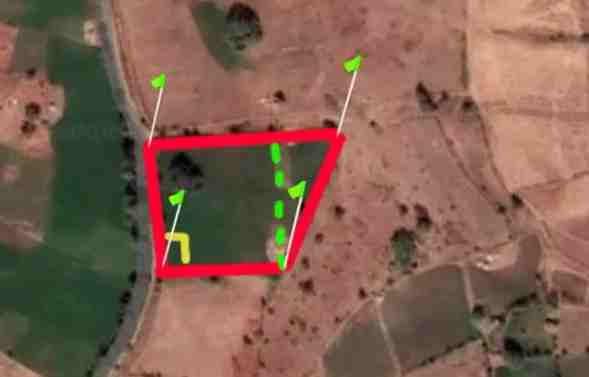We have, Formula

Area of a Trapezium = A = 1/2 x h (a + b)

where h = Height of Trapezium, a = Upper length of trapezium, b = Lower Length of Trapezium

You can also calculate the area of above land by dividing and into two parts in rectangle and triangle which you can see in the figure by the green line.

After that you can again use formula of square and triangle to each other and get total area. How To Measure Irregular Land Area

I hope you understood and you can calculate the area of these types of land. Let us see another type of irregular land which you generally found at the Survey. but don’t worry I will do the numerical of this land also, s let us move forward.

### B) Land having no any side parallel to each other:

This type of land is mostly found in every place of state and country. This is irregular land. In these types of land, all sides are different from each other and also they make different angles with each other.

Surveyors have to calculate these types of land so, often and they get more problems in such types of land to calculate actual area. le us understand with numerical and formula to calculate the area of land.

### Q) Calculate the area of irregular land having dimension given in the figure below:

To calculate these types of land is very easy. to find out the area of these types of land, first of all, burry four pegs or ranging rod at all comers of the land. after that take measurement of all sides of the land. and keep in mind you must take the measurement of any one of the diagonal of plots.

Now, you can see in the figure, total land has divided into two-parts with blue line diagonals and they are triangle in shape.

That’s all, use the formula of triangular land separately and add both to get the total area. isn’t it easy ?

Now, let us move on calculation,

in triangle ABD,

let, BD (a) = 5 meter, AD (b) = 3 meter, AB (c) =4 meter

Again We have, Formula

As we know that, Area of a Scalene Triangle in terms of three sides,

Area of a Triangle = Area = A = √s(s-a)(s-b)(s-c)

where, s = semi-perimeter , a = length of 1st side, b = Length of 2nd side, c = Length of 3rd side

so at first calculate semi-perimeter s = (a+b+c)/2 = (5+3+4)/2 = 6 m

So, Putting all value we get Area of triangle ABD = 6 m2

In the same way,

for triangle BDC,

let, DC (a) = 13 meter, BC (b) = 12 meter, BD (c) = 5 me ter

where, we have (S) = (13+ 12+5)/2= 15 meter.

So, putting all value

we get Area of triangle BDC = 30 m2

Hence total are of land become,

= Area of triangle ABD and triangle BDC

= (6+30) m2

=36 m2

Hence, in this way, we can calculate the area of land which is irregular in shape.

## 5) Polygon Shape land:

Here comes the difficult one, Polygon shape Area of Unevenly shaped site: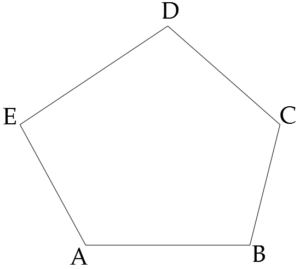With this shape of uneven (polygon) area measurements, we have to divide the shape into as many as possible known geometric shapes like triangle, rectangle etc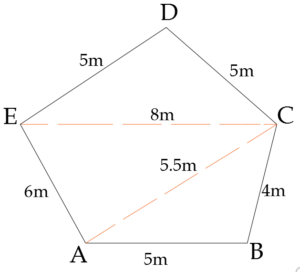Here, we have divided this into 3 triangles (ABC, ACE, ECD) Using Heron’s formula for area calculation.

Area = √s(s-a)(s-b)(s-c)  where s = (a+b+c)/2

Area For ABC (A1):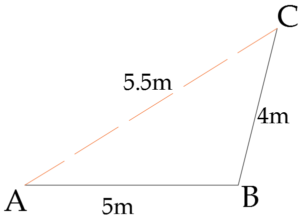S = (a+b+c)/2   (Where AB=a, BC=b, CA=c)

= (5+4+5.5)/2 = 7.25

Area = √s(s-a)(s-b)(s-c) = √7.25(7.25-5)(7.25-4)(7.25-5.5)

A1 = 3.58 m2

Area For ACE (A2):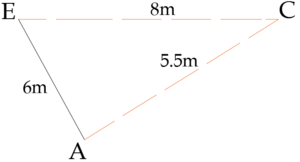S = (a+b+c)/2   (Where AC=a, CE=b, EA=c)

= (5.5+8+6)/2

= 9.75

Area = √s(s-a)(s-b)(s-c) = √9.75(9.75-5.5)(9.75-8)(9.75-6)

Area = A2 = 16.49 m2

Area For ECD (A3):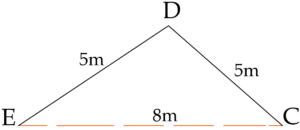S = (a+b+c)/2   (Where EC=a, CD=b, DE=c)

= (8+5+5)/2 = 9

Area = √s(s-a)(s-b)(s-c) = √9(9-8)(9-5)(9-5)

A3 = 12 m2

Total Area = A1+A2+A3 = 3.58+16.49+12 = 32.07 m2

Total Area = 32.07 m2

## Conclusion:

Full article on How to Calculate Land Area | How to Calculate Plot Area | Irregular Land Area Calculator | Land Area Calculation Formula | Land Measurement Formula | How To Measure Irregular Land Area. Thank you for the full reading of this article in “The Civil Engineering” platform in English. If you find this post helpful, then help others by sharing it on social media. If any formula of BBS is missing from this article please tell me in comments.

1.Fre